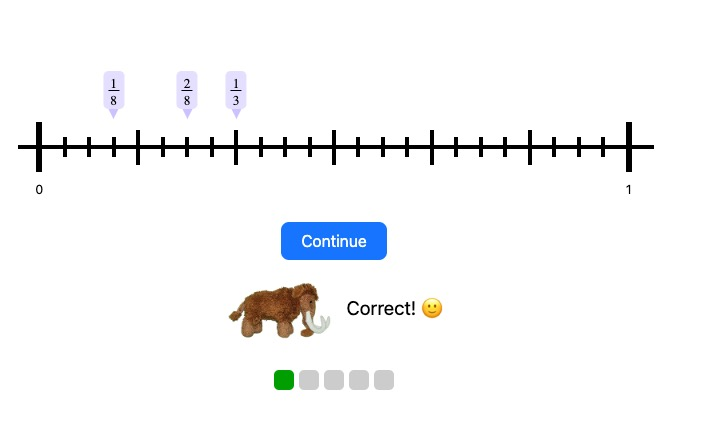MM Practice
×
Multiplication
Division
Place Value
Fractions & Decimals
Measurement
Statistics & Probability
Pre-Algebra
Money
Kindergarten
Geometry

# Order Fractions on a Number Line

## Online practice for grades 3-6

In this activity, you will order three fractions on the number line.

When the fractions to order have different denominators, you will need to find a common denominator for them, using the principles of equivalent fractions. After that, it will be fairly easy to place them on the number line.

Options include fractions with the same or different denominator and the number of problems.